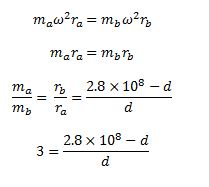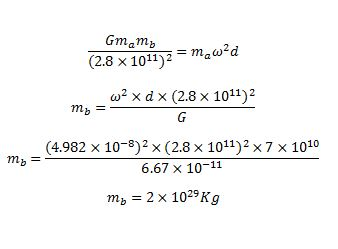Physic Tutorials

# How to solve questions on gravitational field for Cambridge A level and UTME

How to solve questions on gravitational field for Cambridge A level and UTME

Question 1

The earth is four times the size of the moon and the acceleration due to gravity on the earth is 80 times that on the moon. The ratio of the mass of the moon to that of the earth is(gravitational force UTME 2004)

A.  1 : 320                            B. 1 : 1280               C. 1 : 80                                 D. 1 : 4

Solution

size of earth = 4 x size of moon

r(earth) = 4r(m00n)

G of erath = 80 x g of moon

g = GM/r2

GM(earth)/r2(earth) = 80 *GM(moon)/r2(moon)

M(earth)/16r2(moon) = 80 * M(moon)/r2(moon)

M(earth) = 80*16 M(moon)

M(moon) / M(earth) = 1 / 1280

B is the correct option

Question 2

A binary star consists of two stars A and B that orbit one another, as illustrated in Fig belowThe stars are in circular orbits with the centres of both orbits at point P, a distance d from the centre of star A.

i.  The period of the orbit of the stars about point P is 4.0 years. Calculate the angular speed ω of the stars

ii.   The separation of the centres of the stars is 2.8 × 108 km. The mass of star A is MA. The mass of star B is MB.
The ratioMA/MB is 3.0. Determine the distance d.

iii.  Use your answers in (i) and (ii) to determine the mass MB of star B.

May/June 2016 p42

Solutionii

since they orbit at the same point, there centripetal force will be the samecross multiply

3d = 2.8 × 108 – d

3d + d = 2.8 × 108

4d = 2.8 × 108

d = 2.8 × 108 / 4

d = 7 x 10^7 km

iii

To calculate for the mass of star B, You must note that the gravitational force will be equal to the centripetal force for star B to orbit star ARecommended: short note on gravitational field

### Bolarinwa Olajire

A lecturer, Educationist, PhD student at FUNAAB, and a Blogger.